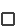## Problem Set

Refer to the Data Analysis subject and answer the following using the default data set.

1. For each individual stock (i.e., set the portfolio weight for the stock being analyzed and zero for all others) record the mean, volatility and JB statistic.

a. Judge whether or not you reject the null hypothesis of normality for each stock.

If returns are described by the normal distribution then return and risk can be completely described by the first two moments, mean and variance (= volatility squared) of the return distribution.

b. If returns deviate from normality classify the third and fourth moments (skewness and kurtosis) for each stock into the following:

Skewness Positive, negative or zero;

Kurtosis Leptokurtic, Platykurtic, and Mesokurtic

Skewness: The measure of asymmetry of data around the sample mean. Negative skewness implies distribution spread is more to the left of the mean than it is to the right, and vice versa for positive skewness. The skewness of a standard normal distribution is zero.

Kurtosis: This moment answers the question as to how outlier prone is the return distribution? You can check for the following behavior:

Leptokurtic distribution --- peaked in center thin in tails (relative to a Standard Normal Distribution Kurtosis is greater than 3).
Platykurtic distribution --- fat in tails low in center (relative to a Standard Normal Distribution kurtosis is less than 3).
Mesokurtic distribution --- Symmetrical normal distribution (Kurtosis equal to 3 for a standard normal distribution)

c. Compute the average of security mean and volatility returns.

2. Select from the drop down menu below plot return histogram, Equally weighted and again record the mean/volatility and JB statistic for this portfolio. Judge whether or not you reject the null hypothesis of normality for this portfolio. Compare the mean and volatility of returns for this naively diversified portfolio to the average of individual security means and volatilities in question 1. Select from the drop down menu below plot return histogram, Equally weighted and again record the mean/volatility and JB statistic for this portfolio. Judge whether or not you reject the null hypothesis of normality for this portfolio. Compare the mean and volatility of returns for this naively diversified portfolio to the average of individual security means and volatilities in question 1.

a. Which differs the most, the mean or volatility?

b. Are these observed differences consistent with what you would predict and why?Refer to the Portfolios Subject and answer the following using the default data set:

3. Construct the (ex post i.e., based on realized returns) efficient portfolio for a very high target return and a normal (e.g., around 10% per annum or less than 1% per month) target return (returns are monthly).

You can do this by clicking anywhere on the efficient portfolio and then recording the risk and return associated with your portfolio. You may also want to copy and paste the portfolio weights into Excel for temporary storage. The return number is beside Target and the risk number is beside Volatility (which is also a monthly volatility for this data set).

a. Inspect the portfolio weights relative to the scatter plot of securities. How is the high target return being achieved by the portfolio weights relative to the normal return portfolio?A naively diversified portfolio is equally weighted. Although ex post it looks like it is easy to dominate this portfolio it is far less easy to do this ex ante.

4. Your goal is to see whether you can find a portfolio on the efficient frontier constructed from 60-months of return data history that dominates the risk and return backtesting performance of the naively diversified portfolio in a backtesting experiment.

Aside: You will discover that Naive diversification performs very well and you have to look to the more stable regions of the efficient frontier to achieve this current objective.Use the FTS Historical Data module to build a spreadsheet of historic price data for a set of stocks or mutual funds of interest to you.

5. By applying Markowitz diversification techniques can you dominate a naively diversified portfolio in backtesting experiments?

Tip: You should first set up the symbols in row 1, columns B, C, D etc., of a spreadsheet in order to use the FTS Historic Data Collection module. To get the correct symbols go to www.yahoo.com, and then Yahoo finance. From the symbol look up you can go to the screener helps such as stock screener, fund screener to identify the potential set of securities you want to work with.